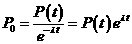Keyah Math Module 12, Level 4

Mathematical Content: Exponential and logarithmic functions, algebraic operations, graphs

# Finding the Age of the Earth: Level 4

Solution to Question 2.

First, solve the basic decay equation for P0,Now let D(t) denote the number of daughter atoms at time t. The number of daughter atoms at time t is equal to the number of parent atoms present initially minus the number of parent atoms at time t, soThis material is based upon work supported by the National Science Foundation under Grant GEO-0355224. Any opinions, findings, and conclusions or recommendations expressed in this material are those of the authors and do not necessarily reflect the views of the National Science Foundation.